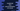# JavaScript program to find out the area and perimeter of a circle# JavaScript practice: Find out the area and perimeter of a circle :

This is a JavaScript practice problem. We will learn how to find the area and perimeter of a circle in JavaScript. We will use one class to solve this problem. With this program, you will get familiar with JavaScript class and object, JavaScript Math class and how to use constants of Math class in a JavaScript program.

## Algorithm :

We need only the radius to calculate both area and perimeter of a circle.

``````Perimeter = 2 * π * radius

So, we need π and radius to calculate both. π is a constant. We can store it in a variable or we can use its value from JavaScript Math module. In this example, we will use the Math module. We will use one class to hold the value of radius and the methods to do these calculations.

## JavaScript program :

``````class Circle {
constructor(r) {
}

getArea() {
}

getPerimeter() {
return (2 * Math.PI * this.radius).toFixed(2);
}
}

### Explanation :

1. Circle class is used to hold the value of the radius. It has one constructor. It takes the radius as an argument and assigns it to the local variable radius.
2. This class also has two methods getArea() and getPerimeter() to get the area and perimeter of a circle for the current radius. toFixed(2) is used to change the calculated value to two decimal places.
3. In this example, we are creating one variable radius(this is a different variable) with value 10.
4. We are creating one Circle object by passing the value of radius to its constructor.
5. Finally, we are printing the area and perimeter of the circle.

It will print the below output :

``````Area for radius 10 is 314.16
Perimeter for radius 10 is 62.83``````

Try to run the example with a different values of radius and drop a comment below if you have any queries.